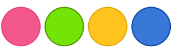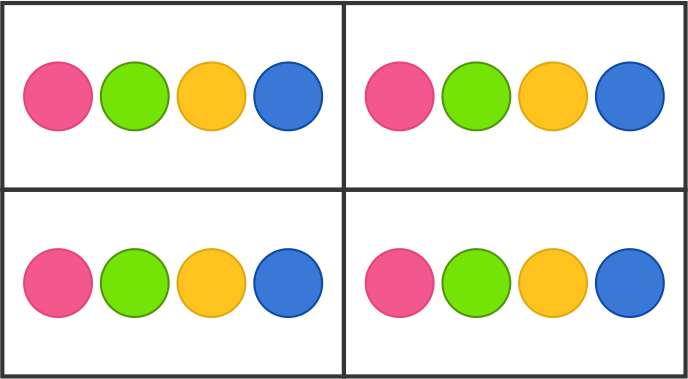# Division - Definition with Examples

The Complete K-5 Math Learning Program Built for Your Child

• 40 Million Kids

Loved by kids and parent worldwide

• 50,000 Schools

Trusted by teachers across schools

• Comprehensive Curriculum

Aligned to Common Core

What is Division?

The division is a method of distributing a group of things into equal parts. It is one of the four basic operations of arithmetic, which gives a fair result of sharing.

The division is an operation inverse of multiplication. If 3 groups of 4 make 12 in multiplication; 12 divided into 3 equal groups give 4 in each group in division.

The main goal of the division is to see how many equal groups or how many in each group when sharing fairly.

For example:

There are 16 balls and 4 boxes, how to put 16 balls into four equal sized boxes?So, 16 divided by 4 =?Therefore, 4 balls should be kept in each box.

Mathematical notation for division

There are various signs which can be used to indicate division such as ÷, /.

For example:

• 8 ÷ 2
• 8/2

Special names for each character in Division

Each part involved in a division equation has a special name.

Dividend ÷ divisor = quotient

Dividend: The dividend is the number that is being divided in the division process.

Divisor:The number by which dividend is being divided by is called divisor.

Quotient: A quotient is a result obtained in the division process.

18    ÷   3    =    6Dividend        divisor         quotient

So, in the above process where we divided 16 balls into 4 equal groups;

The dividend is 16, the divisor is 4 and thus the quotient is 4.

Introduction to the remainder

The remainder is the portion of the dividend that is left over after division. For example, on dividing 83 by 2, there is a leftover of 1.

It means, 83 ÷ 2  = 41 and  r =1,

Here, ‘r’ is remainder.

• When dividing something by 1, the answer will always be the original number. It means if the divisor is 1, the quotient will always be equal to the dividend such as 10 ÷ 1= 10.

• Division by 0 is undefined.

• The division of the same dividend and divisor is always 1. For example: 4 ÷ 4 = 1.

 Fun Facts about the division The oblique bar used as a sign in division process was introduced by De Morgan in 1845.

Won Numerous Awards & Honors